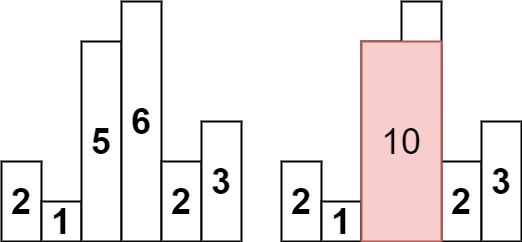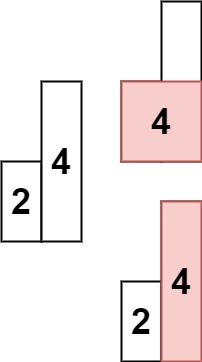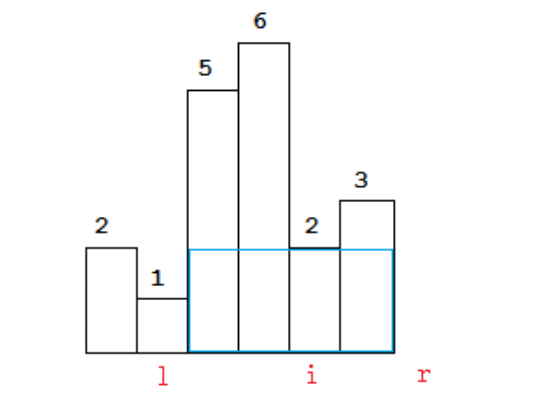# 84. Largest Rectangle in Histogram

Given an array of integers heights representing the histogram’s bar height where the width of each bar is 1, return the area of the largest rectangle in the histogram.

## EX

Example 1:Input: heights = [2,1,5,6,2,3]
Output: 10
Explanation: The above is a histogram where width of each bar is 1.
The largest rectangle is shown in the red area, which has an area = 10 units.


Example 2:Input: heights = [2,4]
Output: 4


## Constraints:

1 <= heights.length <= 105 0 <= heights[i] <= 104

# V1-暴力解法

## 解法

    /**
* 最大的长方形面积
*
* 1. 首先直接暴力计算一遍
*
*
* @param heights 高度数组
* @return 结果
*/
public int largestRectangleArea(int[] heights) {
// 最大值
int max = Integer.MIN_VALUE;

for(int i = 0; i < heights.length; i++) {
for(int j = i; j < heights.length; j++) {
// 对比
int width = j - i + 1;

// 应该是从 i..j的最小值
int minHeight = min(heights, i, j);

max = Math.max(width * minHeight, max);
}
}

return max;
}

private int min(int[] nums,
int i,
int j) {
int min = Integer.MAX_VALUE;

for(int k = i; k <= j; k++) {
min = Math.min(min, nums[k]);
}
return min;
}


# V2-改进算法

## 思路

For any bar i the maximum rectangle is of width r - l - 1 where r - is the last coordinate of the bar to the right with height h[r] >= h[i] and l - is the last coordinate of the bar to the left which height h[l] >= h[i]

So if for any i coordinate we know his utmost higher (or of the same height) neighbors to the right and to the left, we can easily find the largest rectangle:


int maxArea = 0;
for (int i = 0; i < height.length; i++) {
maxArea = Math.max(maxArea, height[i] * (lessFromRight[i] - lessFromLeft[i] - 1));
}

The main trick is how to effectively calculate lessFromRight and lessFromLeft arrays.

The trivial solution is to use O(n^2) solution and for each i element first find his left/right heighbour in the second inner loop just iterating back or forward:


for (int i = 1; i < height.length; i++) {
int p = i - 1;
while (p >= 0 && height[p] >= height[i]) {
p--;
}
lessFromLeft[i] = p;
}

The only line change shifts this algorithm from O(n^2) to O(n) complexity: we don't need to rescan each item to the left - we can reuse results of previous calculations and "jump" through indices in quick manner:


while (p >= 0 && height[p] >= height[i]) {
p = lessFromLeft[p];
}


## 一点额外的解释

### r l

The meaning of r and l is somewhat confusing, to put them more accurately:

l: the first coordinate of the bar to the left with height h[l] < h[i].

r: the first coordinate of the bar to the right with height h[r] < h[i].### p = lessFromLeft[p]

Here's the intuition to understand p = lessFromLeft[p]; :

Consider the test case
indices.......... : 0 1 2 3 4 5 6 7 8 9 10 11
heights.......... : 4 9 8 7 6 5 9 8 7 6 5 4.
lessFromLeft :-1 0 0 0 0 0 5 5 5 5 . .

In this, when we reach 5 at index 10, we start searching for idx=9, i.e. p points at 6.
6 > 5.
Now, we want something which is smaller than 5, so it should definitely be smaller than 6. So 6 says to 5:

I've already done the effort to find the nearest number that's smaller than me and you needn't traverse the array again till that point. My lessFromLeft points at index 5 and all the elements between that and me are greater than me so they are surely greater than you. So just jump to that index and start searching from there.

So you next p directly points at idx = 5, at value 5.

There, this 5 again says the same statement to current 5 and asks it to jump directly to idx = 0. So in the second iteration itself, our search has reached idx=0 and that's our answer for the current element.

Similarly, for the next element 4, it'll take 3 steps.

And for all the elements following 4, if they are greater than 4, their search will stop at 4 itself.

Bottomline: we are not traversing the array again and again. it's O(n).


indices.......... : 0 1 2 3 4 5 6 7 8 9 10 11
heights.......... : 4 9 8 7 6 5 9 8 7 6 5 4.
lessFromLeft :-1 0 0 0 0 0 5 5 5 5 . .


6 > 5。

## 完整实现

public static int largestRectangleArea(int[] height) {
if (height == null || height.length == 0) {
return 0;
}
int[] lessFromLeft = new int[height.length]; // idx of the first bar the left that is lower than current
int[] lessFromRight = new int[height.length]; // idx of the first bar the right that is lower than current
lessFromRight[height.length - 1] = height.length;
lessFromLeft = -1;

for (int i = 1; i < height.length; i++) {
int p = i - 1;

while (p >= 0 && height[p] >= height[i]) {
p = lessFromLeft[p];
}
lessFromLeft[i] = p;
}

for (int i = height.length - 2; i >= 0; i--) {
int p = i + 1;

while (p < height.length && height[p] >= height[i]) {
p = lessFromRight[p];
}
lessFromRight[i] = p;
}

int maxArea = 0;
for (int i = 0; i < height.length; i++) {
maxArea = Math.max(maxArea, height[i] * (lessFromRight[i] - lessFromLeft[i] - 1));
}

return maxArea;
}


（1）一个是对于最大矩形的理解

（2）O(N) 算出 lessFromRight/lessFromLeft